## Computing matrix inverse mod p in Matlab

The command “inv" in Matlab compute the inverse of a matrix over the real numbers or complex numbers. If we need to compute the inverse of a matrix, whose entries are drawn fromfor some prime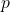, then certainly we cannot apply the function inv directly. The result obtained by Matlab command inv is a matrix over real numbers, but we want a matrix whose entries are integers between 0 to.

Instead, we can obtain the adjugate matrix, or the adjoint of a matrix, provided that the determinant of the matrix does not cause any integer overflow. For a matrixwith integer entries, the adjoint is defined as the transpose of the cofactor matrix, and the entries of it are all integers. I cannot find a Matlab command for calculating the adjoint of a matrix, but we can calculate it indirectly by inv(A)*det(A). Here, det(A) is the determinant of matrixand is an integer.

The correct answer is simply the product of  inv(A)*det(A) and the multiplicative inverse of det(A) mod p.

The following program illustrate the procedure. A is a random matrix with entries between 0 and. B is the multiplicative inverse of A mod.

p = 11; % a prime number
A = floor(rand(4,4)*p)  % a randomly generated integer matrix

determinant = round(mod(det(A),p));

if determinant == 0
disp('matrix is singular')
else
[d r s] = gcd(determinant,p);
B =  mod(round(inv(A)*det(A)*r),p)  % B is the inverse of A mod p
mod(A*B,p)  % check that B times A mod p is the identity matrix
end;

The sample output is

A =

3     5     6     6
6     8     2     9
5     1     6     5
7     5     0     4

B =

10     0     1     3
2     3     8     5
8     4     0     1
2    10     2     8

ans =

1     0     0     0
0     1     0     0
0     0     1     0
0     0     0     1

## Lecture notes for ENGG2420

Some lecture notes I typed for the course ENGG2420B “Complex analysis and Differential Equations for Engineering”:

## ENGG2420B

I am currently teaching a math. course on complex analysis and differential equations.

This year, I adopt the Piazza e-learning platform. The Piazza page of the course is here.

## What is dx?

Calculus teacher: It reminds you that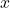is the variable in the integration.

Private math. tutor: Points will be deducted if one forgets to write it down.

Engineer: It is an infinitesimally small.

Teacher of advanced calculus: It is the differential of the function.

Measure theorist: We usually writeinstead.

Differential geometer: It is a smooth function mapping a point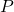in a manifold to an element in the cotangent space at.

Algebraic geometer: Let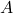be a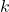-algebra over a field.is the equivalent classin the quotient, where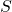is the-submodule ofgenerated by elements of the form. In general,is.